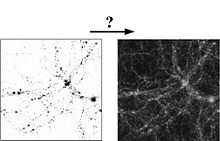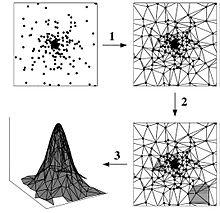# Delaunay tessellation field estimator

﻿
Delaunay tessellation field estimatorThe reconstruction of a density field from a discrete set of points sampling this field.

The Delaunay tessellation field estimator (DTFE) is a mathematical tool for reconstructing a volume-covering and continuous density or intensity field from a discrete point set. The DTFE has various astrophysical applications, such as the analysis of numerical simulations of cosmic structure formation, the mapping of the large scale structure in the universe and improving computer simulation programs of cosmic structure formation. It has been developed by Willem Schaap and Rien van de Weijgaert. The main advantage of the DTFE is that it automatically adapts to (strong) variations in density and geometry. It is therefore very well suited for studies of the large scale galaxy distribution.

## Method

The DTFE consists of three main steps:Overview of the DTFE procedure.

### Step 1

The starting point is a given discrete point distribution. In the upper left-hand frame of the figure a point distribution is plotted in which at the center of the frame an object is located whose density diminishes radially outwards. In the first step of the DTFE the Delaunay tessellation of the point distribution is constructed. This is a volume-covering division of space into triangles (tetrahedra in three dimensions), whose vertices are formed by the point distribution (see figure, upper right-hand frame). The Delaunay tessellation is defined such that inside the interior of the circumcircle of each Delaunay triangle no other points from the defining point distribution are present.

### Step 2

The Delaunay tessellation forms the heart of the DTFE. In the figure it is clearly visible that the tessellation automatically adapts to both the local density and geometry of the point distribution: where the density is high, the triangles are small and vice versa. The size of the triangles is therefore a measure of the local density of the point distribution. This property of the Delaunay tessellation is exploited in step 2 of the DTFE, in which the local density is estimated at the locations of the sampling points. For this purpose the density is defined at the location of each sampling point as the inverse of the area of its surrounding Delaunay triangles (times a normalization constant, see figure, lower right-hand frame).

### Step 3

In step 3 these density estimates are interpolated to any other point, by assuming that inside each Delaunay triangle the density field varies linearly (see figure, lower left-hand frame).

## Applications

### An atlas of the nearby universe

One of the main applications of the DTFE is the rendering of our cosmic neighborhood. Below the DTFE reconstruction of the 2dF Galaxy Redshift Survey is shown, revealing an impressive view on the cosmic structures in the nearby universe. Several superclusters stand out, such as the Sloan Great Wall, the largest structure in the universe known to date.

### Numerical simulations of structure formation

Most algorithms for simulating cosmic structure formation are particle hydrodynamics codes. At the core of these codes is the smoothed particle hydrodynamics (SPH) density estimation procedure. Replacing it by the DTFE density estimate will yield a major improvement for simulations incorporating feedback processes, which play a major role in galaxy and star formation.

### Cosmic velocity fieldDTFE velocity field reconstructions of superclusters and voids in the large scale galaxy distribution.

The DTFE has been designed for reconstructing density or intensity fields from a discrete set of irregularly distributed points sampling this field. However, it can also be used to reconstruct other continuous fields which have been sampled at the locations of these points, for example the cosmic velocity field. The use of the DTFE for this purpose has the same advantages as it has for reconstructing density fields. The fields are reconstructed locally without the application of an artificial or user-dependent smoothing procedure, resulting in an optimal resolution and the suppression of shot noise effects. The estimated quantities are volume-covering and allow for a direct comparison with theoretical predictions.

### Evolution and dynamics of the cosmic web

The DTFE has been specifically designed for describing the complex properties of the cosmic web. It can therefore be used to study the evolution of voids and superclusters in the large scale matter galaxy distribution.

Wikimedia Foundation. 2010.

### Look at other dictionaries:

• Delaunay triangulation — A Delaunay triangulation in the plane with circumcircles shown In mathematics and computational geometry, a Delaunay triangulation for a set P of points in the plane is a triangulation DT(P) such that no point in P is inside the circumcircle of… …   Wikipedia

• List of mathematics articles (D) — NOTOC D D distribution D module D D Agostino s K squared test D Alembert Euler condition D Alembert operator D Alembert s formula D Alembert s paradox D Alembert s principle Dagger category Dagger compact category Dagger symmetric monoidal… …   Wikipedia